Students can access the CBSE Sample Papers for Class 11 Chemistry with Solutions and marking scheme Term 2 Set 2 will help students in understanding the difficulty level of the exam.

## CBSE Sample Papers for Class 11 Chemistry Standard Term 2 Set 2 with Solutions

Max. Marks: 40
Time: 2 Hours

General Instructions:

• There are 12 questions in this question paper xoith internal choice.
• Section A: Q. No. 1 to 3 are very short answer questions carrying 2 marks each.
• Section B: Q. No. 4 to 11 are short answer questions carrying 3 marks each.
• Section C: Q. No. 12 is case based question carrying 5 marks.
• All questions are compulsory.
• Use of log tables and calculators is not allowed.

Section-A

Question 1.
Arrange the following in the increasing order of their property indicated (Any 2):(ii) n-butane, 2-methylbutane, n-pentane, 2,2-dimethylpropane (boiling points)
(iii) H2C=CH2, (CH3)2C = CH2 ,CH3– CH= CH2, CH3 -CH= CH -CH2 – CH3
(i) CH3Br does not have any β-carbon so it would not show any reaction. More the number of β-substituents
(alkyl groups), more stable alkene will be formed on β-elimination and more will be the reactivity. Thus, the increasing order of reactivity of the rate of β- elimination with aic. KOH is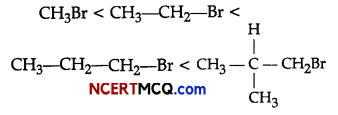(ii) n-butane < 2,2-dimethyipropane < 2-methyl butane < n-pentane. As the number of carbon atoms in the chain increases, boiling point increases. Boiling point decreases with increase in branching.

(iii) The stability of the intermediate carbocation determines the reactivity towards electrophilic addition reactions. Due to the inductive effect (+1) of alkyl group, the bigger is the attached alkyl group, the more stable is the intermediate ion. Thus, the order of stability of carbocation is: Primary carbocation(1°) < Secondary carbo cation (2°) alkenes towards electrophilic addition reactions increases in the order:
H2C=CH2, <CH3-CH= CH2 <CH3-CH=CH-CH2-CH3 <(CH3)2C= CH2Question 2.
Out of the two pairs of compounds given below, identify the compound in each of the pairs which has Group 13 element in more stable oxidation state. Mention the nature of bonding too.
(i) TlCl3,TlCl
(ii) AlCl3, AlCl
(i) TlCl has more stable oxidation state than TlCl3 because on moving down the group due to inert pair effect, lower oxidation state is more stable. TlCl is ionic in nature.

(ii) AlCl3 has more stable oxidation state than AlCl because it does not display inert pair effect. The anhydrous state of AlCl3 is covalent while its aqueous state is ionic in nature.

Question 3.
(i) Benzene is extraordinarily stable though it contains three double bonds.
(ii) Toluene on Friedel-Crafts methylation gives o- and p-xylene.
(i) Benzene is a hybrid of resonating structures given as:All six carbon atoms in benzene are sp2 hybridized. The two sp2 hybrid orbitals of each carbon atom overlap with the sp2 hybrid orbitals of adjacent carbon atoms to form six sigma bonds in the hexagonal plane.
The remaining sp2 hybrid orbital on each carbon atom overlaps with the s-orbital of hydrogen to form six sigma C-H bonds. The remaining unhybridized p-orbital of carbon atoms has the possibility of forming three it bonds.The six π-electrons are delocalized and can move freely about the six-carbon nuclei. Even after the presence of three double bonds, these delocalized π-electrons stabilise benzene.
(ii) Methyl (-CH3) group attached to benzene ring increases electron density to benzene ring at ortho and para-positions due to its electron releasing nature (i.e., + R effect), So, toluene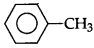on Friedel- Crafts methylation gives o-and p-xylene.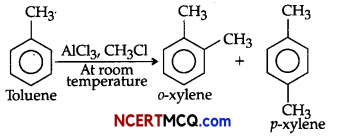Section – B

Question 4.
Account for the following:
(i) The hydroxides and carbonates of sodium and potassium are easily soluble in water while the corresponding salts of magnesium and calcium are sparingly soluble in water.
(ii) Potassium and caesium rather than lithium are used in photoelectric cells.
(iii) Lithium is a strong reducing agent in aqueous solution.
OR
Write balanced equations for chemical reactions between the following:
(i) Na2O2 and water
(ii) KO2 and water
(iii) Na2O and CO2.
(i) The solubility of a salt in water depends upon their lattice energy and hydration energy.
In the case of hydroxide and carbonates of sodium and potassium, their hydration energy is more than their lattice energy.

Therefore, they dissolve in water. In the case of hydroxides and carbonates of magnesium and calcium, their hydration energy is less than their lattice energy. Therefore, they are sparingly soluble.

(ii) Due to low ionisation enthalpy, potassium and caesium eject electrons when exposed to light while lithium has the highest ionization enthalpy due to which it cannot be used in photoelectric cells.

(iii) The ionic size of lithium is small so it has highest hydration enthalpy. Ionisation energy of Li is highest among alkali metals but hydration enthalpy predominates over ionisation enthalpy, Thus, lithium has strong reducing power in aqueous solution.
Or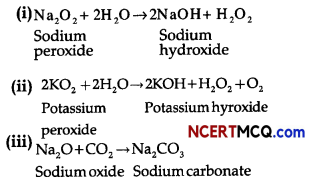Question 5.
(i) If two substances are in equilibrium in a reversible reaction and if the concentration of each reactant is reduced to half, then how the equilibrium constant will be affected?
(ii)
(a) Fizz is observed when soda water bottle is opened. Why?
(b) For an exothermic reaction, what happens to the equilibrium constant if temperature is increased?
OR
(i) A sparingly soluble salt having general formula Ap+x Bq-y and molar solubility S is in equilibrium with its saturated solution. What will be the relationship between solubility and solubility product of such salt?
(ii) (a) State Le-Chatelier’s principle.
(b) For the given reaction CO(g) + 2H2(g) ⇌ CH3OH(g), ∆rH° = – 92 kj/mol, predict the direction of the reaction where:
(1) pressure is doubled
(2) temperature is doubled.
(i) The equilibrium constant of a reaction is the ratio of the concentration of products to the concentration of reactants at equilibrium. It is a measure of the extent of the reaction. It is independent of the rate of reaction and initial concentrations of reactants and products but depends on the temperature and pressure. Hence, Keq is not affected by change in concentration of reactants and products.

(ii)
(a) An unopened soda water bottle is virtually bubble-free because the pressure inside the bottle keeps the carbon dioxide dissolved in the liquid. When it is opened, pressure is released and the gas bubbles are allowed to wiggle free from the liquid and rise to the surface in the form of fizz.

(b) Equilibrium constant, K = $$\frac{\mathrm{K}_{f}}{\mathrm{~K}_{b}}$$
In exothermic reaction, with the increase in temperature, equilibrium constant for backward reaction (Kb) increases much more than Kf. Thus, equilibrium constant (K) decreases with increase in temperature for an exothermic reaction.

OR
(i) A sparingly soluble salt having general formula Axp+’ Byq-, its molar solubility is S mol L-1
Then, Axp+’ Byq- ⇌ xAp+(aq) +yBq-(aq)
S moles of AxBy dissolve to give x moles of Ap+ and y moles of Bq-.
Therefore, solubility product
Ksp= [Ap+]x[Bq-]y
= [xS]x[yS]y
= xxyySx+y

(ii) (a) According to Le – Chatelie’s principle, “A change in any of the factors that determine the equilibrium conditions of a system will cause the system to change in such a manner so as to reduce or to counteract the effect of the change”.

(b) CO(g) + 2H2(g) ⇌ CH3OH(g),
ΔrH0 = -92 kJ/mol

(1) When pressure is doubled, the equilibrium will shift in the direction where volume decreases i.e. forward direction.
(2) When temperature is doubled, the equilibrium will shift in backward direction because it is an exothermic reaction ΔH <O.Question 6.
Account for the following:
(i) PbCl4 is less stable than SnCl4 but PbCl2 is more stable than SnCl2.
(ii) Carbon shows maximum catenation in Group 14 elements.
(iii) Diamond is covalent, yet it has high melting point.
(i) Stability of +4 oxidation state decreases down the group while that of +2 oxidation state increases due to inert pair effect. Thus, PbCl2 and SnCl4 are more stable than PbCl4 and SnCl2 respectively.
(ii) Due to strong C-C bond, its bond dissociation energy is the highest among Group 14 elements.
(iii) Diamond has three dimensional network structure involving strong C-C bonds. These bonds are difficult to break. Hence, the melting point of diamond is very high.

Question 7.
Determine the degree of ionisation and pH of a 0.05 M of ammonia solution. The ionisation constant of ammonia is 1.77 x 10-5. Also calculate the ionisation constant of conjugate add of ammonia.
Given,
Kb = 1.77 x 10-5
C =0.05 M
The ionisation of NH3 in water is represented by the equation:where, α = Degree of ionization
C = concentration of solution
Ionization constant of ammonia,

Kb$$\frac{\left[\mathrm{NH}_{4}^{+}\right]\left[\mathrm{OH}^{-}\right]}{\left[\mathrm{NH}_{3}\right]}$$
or α = $$\sqrt{\frac{\mathrm{K}_{\mathrm{b}}}{\mathrm{C}}}$$
= $$\frac{\mathrm{C} \alpha \cdot \mathrm{C} \alpha}{\mathrm{C}(1-\alpha)}$$
= $$\frac{\mathrm{C} \alpha^{2}}{(1-\alpha)}$$

The value of α is small, so, the equation can be simplified by neglecting a in comparison to 1 in the denominator on right hand side of the equation.
∴ Kb = Cα2
or α = $$\sqrt{\frac{\mathrm{K}_{\mathrm{b}}}{\mathrm{C}}}$$
= $$\sqrt{\frac{1.77 \times 10^{-5}}{0.05}}$$
= 0.018

[OH] = Cα
= 0.05 x 0.018
= 9.4 x 10-4 M
∵ Ionisation product of water,
[H+] [OH] = Kw
[H+] = $$\frac{\mathrm{K}_{\mathrm{w}}}{\left[\mathrm{OH}^{-}\right]}=\frac{10^{-14}}{9.4 \times 10^{-4}}$$
= 1.06 x 10-11M
Now, pH=-log10[H+]
= -log (1.06 x 10-11)
= -log 1.06 + 11 log 10
= -0.0253 + 11
= 10.9747

Now, using the relation for conjugate acid base pair,
Ka x Kb = Kw
Ka = $$\frac{\mathrm{K}_{\mathrm{w}}}{\mathrm{K}_{\mathrm{b}}}$$
= $$\frac{10^{-14}}{1.77 \times 10^{-5}}$$
=5.64 x 10-10Question 8.
Observe the figure given below and answer the questions that follow:Pressure vs volume graph for a monoatomic ideal gas taken through a cycle of expansion and compression is shown in the figure.
(i) What will be the value of ΔH for the cycle as a whole?
(ii) Define standard enthalpy of formation.
(iii) Define state function with examples.
(i) The value of H for the cycle as a whole will be zero because it is a state function.
(ii) Standard enthalpy of formation (ΔfH0) is defined as the standard enthalpy change for the formation of one mole of a compound from its elements in their most stable states of aggregation.

(iii) A state function is a property whose value does not depend on the path taken to reach that specific value. For example: Pressure volume, enthalpy change and internal energy.

Question 9.
What happens when following conversion occurs:
(i) Alkali metals are heated in the air.
(ii) When sodium is dissolved in liquid ammonia.
(iii) Metal carbonates are heated.
OR
(i) What is the oxidation state of K in KO2?
(ii) Complete the following reactions:
8 LiH+ Al2Cl6 → A + 6LiCl
A+BeCl2 → BL+LiCl+AlCl3
(i) The alkali metals on heating in air form various oxides. Lithium forms monoxide, sodium forms peroxide and potassium forms superoxide.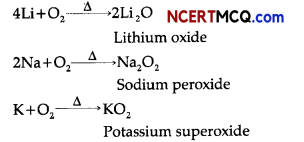(ii) Alkali metals like sodium dissolve in liquid ammonia to give deep blue solution forming ammoniated electrons which absorb energy in visible region of light and impart blue colour to the solution.
M+(x+y)NH3 → [M(NH3)x +2[e(NH3)y] Ammoniated electron

(iii) Metal carbonatos decompose on heating to givemetal oxide and carbon dioxide.OR
(i) Let, oxidation state of K = x
∴ KO2 contains superoxide ion i.e., O2
x + (-1) = O
x-1 =0
x=+1

∴ The oxidation state of K in KO2 is +1.
(ii) 8LiH + Al2Cl6 → 2LiAlH4 + 6LiCl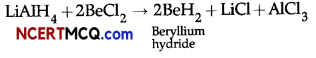A is LlAlH4 and B is BeH2.Question 10.
The combustion of one mole of benzene takes place at 298 K and 1 atm. After combustion, CO2(g) and
H2O(l) are produced and 3267.0 kj of heat is liberated. Calculate the standard enthalpy of formation, ΔfH° of benzene. Standard enthalpies of formation of CO2(g) and H2O(l) are – 393.5 kj mol-1 and – 285.83 kj mol-1 respectively.
Chemical reaction for the formation of benzene,
6C(graphite,s) + 3H2(g) → C6H6(l), ΔfH° =? ……………………. (i)

Enthalpy of combustion of 1 mol of benzene is:
C6H6(l) + $$\frac{15}{2}$$O2(g) → 6CO2(g) + 3H2O(l);
ΔcH°= 3267 kJ mol-1 ………………………………. (ii)

Enthalpy of formation of 1 moI of CO2(g)
C(graphite,s) + O2(g) → CO2(g);
ΔfH° = -393.5 kJ mol-1 …………………………………………… (iii)

Enthalpy of formation of 1 mol of H2O(l) is:
H2(g) + $$\frac{1}{2}$$O2(g) → H2O (l)
ΔfH° = —285.83 kJ mol-1 ………………………………. (iv)

Multiplying eq. (iii) by 6 and eq. (iv) by 3, we get
6C(graphite,s) + 6O2(g) → 6CO2(g)
ΔfH° = —2361 kJ mol-1 ……………………………… (v)

3H2 (g) + $$\frac{3}{2}$$O2 (g) → 3H2O(l);
ΔfH° = -857.49 kJ mol-1 ………………………………. (vi)

On adding above equations, (y) and (vi), we get
6C(graphite,s) + 3H2(g) + $$\frac{15}{2}$$ O2(g) → 6CO2(g) + 3H2O(l);
ΔfH°= -3218.49 kJ mol-1 ……………………………….. (vii)

On reversing eq. (ii), we get
6CO2(g) + 3H2O(l) → C6H6(l) + $$\frac{15}{2}$$ O2(g);
ΔfH° = 3267 kJ mol-1 ………………………………… (viii)

On adding equation (vii) and (viii), we get
6C(graphite,s) + 3H2(g) → C6H6(l);
ΔfH = 48.51 kJ mol-1
∴ Standard enthalpy of formation of benzene
fH°) = 48.51 kJ mol-1

Question 11.
(i) Calculate the temperature of 4.0 moles of a gas occupying volume of 5 dm3 at pressure of 3.32 bar.
[R = 0.083 bar dm3 K-1 mol-1]
(ii) Calculate the total number of electrons present in 1.4 g of dinitrogen gas. BD
(iii) Write the conditions in terms of temperature and pressure, under which all gases obey Charle’s law.
On the basis of the figure given below, answer the following questions: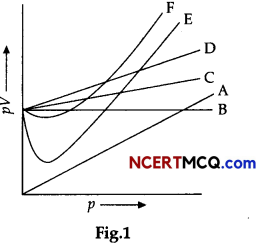(i) Which curve in figure represents the curve of ideal gas?
(ii) State the law followed by the curve B.
(iii) What are the conditions on the application of which a gas deviates from ideal behaviour?
(i) Given,
P =3.32 bar,
V = 5 dm3
R = 0.083 bar dm3 K-1 mol-1
n =4 moIes
By Ideal gas equation,
PV = nRT

T = $$\frac{\mathrm{PV}}{n \mathrm{R}}=\frac{3.32 \times 5}{4 \times 0.083}=\frac{16.6}{0.332}$$
T = 50 k
(ii) No. of moles in 1.4 g of N2 gas = $$\frac{\text { Given mass }}{\text { Molar mass }}$$
= $$\frac{1.4}{28}=\frac{1}{20}$$ = 0.05 mol

Total number of electrons present in 0.05 moles of N2,
= 0.05 x 6.02 x 1023 x 14
= 4.214 x 1023 electrons

(iii) All gases obey Charle’s law at very low pressure and high temperature.
OR
(i) Curve B represents for ideal gas. For ideal gas, pV = constant is represented by straight line. Therefore, only B represents the curve of ideal gas.

(ii) The ideal gas follows Boyle’s law. For ideal gas, PV = constant represented by straight line. Therefore, Boyle’s law states that the pressure (p) of a given quantity of gas varies inversely with its volume (V) at constant temperature i.e., in mathematical expression, pV = k, a constant.

(iii) Gases deviate from ideal behaviour under high pressure and low temperatures because the intermolecular forces come into play as gas particles come close to each other by action of high pressure and low temperature.Section – C

Question 12.
Hydrocarbons are of different types. Saturated hydrocarbons contain carbon-carbon and carbon- hydrogen single bonds. Unsaturated hydrocarbons contain carbon-carbon multiple bonds – double bonds, triple bonds or both. Aromatic hydrocarbons are a special type of cyclic compounds that are also known as ‘arenes’. Most of such compounds were found to contain benzene ring.

Now, the name is applied to all the ring systems whether or not having benzene ring, possessing planarity, complete delocalisation of the electrons in the ring, following Huckel rule. Aromatic hydrocarbons are non¬polar molecules and are usually colourless liquids or solids with a characteristic aroma. Arenes are characterised by electrophilic substitution reactions.
(i) Ethyne on passing through red hot iron tube at 873 K undergoes cyclic polymerisation. Name the product formed in this reaction.
(ii) Write the chemical reaction for conversion of benzene into BHC.
(iii) Examine the following and select the aromatic structures.
img
(iv) How does the presence of halogen atom affect the reactivity of benzene ring towards electrophilic substituition reaction?
OR
Write name and structure of any two arenes.